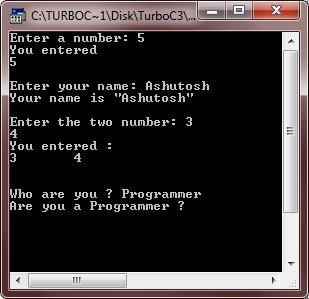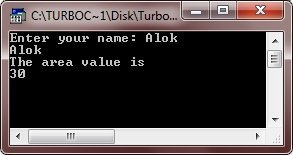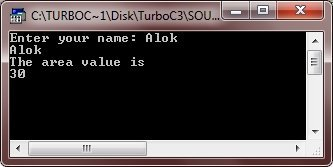# C Constants

Constants refer to a fixed values that the program may not alter. Constants can be of any of the basic data types. The style each constant is represented simply depends upon its type.

Character constants are enclosed in between single quotes. For example, 'a', 'c', and '%' are character constants. String constants are enclosed in between double quotes. For example, "str", "anish", and also "a" (is a string constant containing only one character) are string constants.

Following table lists some of the examples of constants:

Data Type Constant Example
int 1, 23, 450, -32
long int 25000L, -45L
unsigned int 1000U, 345u, 20000U
float 123.32F, 5.3e-4f
double 124.32, 1.0, -0.943234
long double 1001.2L

Here are examples of hexadecimal and octal constants:

```int hex = 0x80;    // 128 in decimal
int oct = 012;    // 10 in decimal```

Here we have listed number conversion programs that you can go for:

## Backslash Character Constants

Enclosing character constants in single quotes works for most printing characters. Here the following table lists the backslash character constants:

Backslash Character Constant Name
\b Backspace
\f Form feed
\n Newline
\r Carriage return
\t Horizontal tab
\' Single quote
\" Double quote
\\ Backslash
\v Vertical tab
\? Question mark
\N Octal constants (here N is an octal constant)

### Backslash Character Constants Example

Here is an example program using backslash character constants:

```/* C Constants - Backslash Character Constants Program */

#include<stdio.h>
#include<conio.h>
#include<stdlib.h>
void main()
{
int num1, num2, num3;
char str1, str2;
clrscr();

printf("Enter a number: ");
scanf("%d", &num1);
printf("You entered\n%d", num1);

fflush(stdin);
gets(str1);

fflush(stdin);
printf("\n\nEnter the two number: ");
scanf("%d%d", &num2, &num3);
printf("You entered :\n%d\t%d\n", num2, num3);

fflush(stdin);
printf("\n\nWho are you ? ");
gets(str2);
printf("Are you a %s\?", str2);

getch();
}```

Here is the sample run of the above C program:## C #define Preprocessor

Here is the general form to use #define preprocessor to define a constant in C:

`#define identifier value`

Here is an example program illustrating the #define preprocessor:

```/* C Constants = This program demonstrates
* the use of #define preprocessor */

#include<stdio.h>
#include<conio.h>

#define LENGTH 5
#define NEWLINE '\n'

void main()
{
int cal_area;
char str;
clrscr();

gets(str);
printf("%s%c", str, NEWLINE);
printf("The area value is %c%d", NEWLINE, cal_area);

getch();
}```

Here is the sample run of the above C program:## C const Keyword

You can use the const prefix to declare constants with a specific type. Here is the general form to declare constants using the keyword const:

`const type variable = value;`

Here is an example program demonstrating the const keyword in defining the constants in C language:

```#include<stdio.h>
#include<conio.h>
void main()
{

const int  LENGTH = 6;
const char NEWLINE = '\n';
int cal_area;
char str;
clrscr();

gets(str);
printf("%s%c", str, NEWLINE);
printf("The area value is %c%d", NEWLINE, LENGTH*BREADTH);

getch();
}```

Following is the sample output of the above C program:### Number Conversion Programs

Here are the list of complete number conversion programs that you can go for:

Tools
Calculator